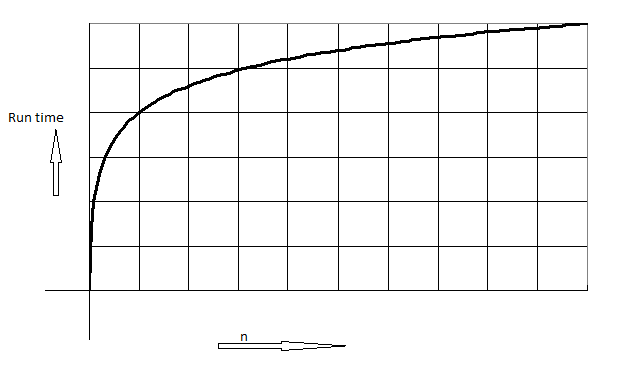# graph binary search for size and time c++ //System Libraries #include <iostream> #include <string> #include <cstdlib> #include <ctime> #include <iomanip> #include <alg...

graph binary search for size and time

c++

//System Libraries
#include <iostream>
#include <string>
#include <cstdlib>
#include <ctime>
#include <iomanip>
#include <algorithm>
using namespace std;
//User Libraries

//Global Constants, no Global Variables are allowed

//Math/Physics/Conversions/Higher Dimensions - i.e. PI, e, etc...

//Function Prototypes

//Execution Begins Here!
int main(int argc, char** argv) {
int n, i, arr, search, first, last, middle,count=0,count_in,tot;
clock_t start, end;
float duration;
cout<<"Enter total number of elements :";
cin>>n;
cout<<"Enter numbers";
for (i=0; i<n;i++)
cin>>arr[i];
cout<<"Enter a number to find :";
cin>>search;
first = 0;
last = n-1;
start = clock();
middle = (first+last)/2;
while (first <= last)
{
count++;
if(arr[middle] < search)
first = middle + 1;
else if(arr[middle] == search)
{
break;
}
else
last = middle - 1;
count_in++;
middle = (first + last)/2;
}
if(first > last)
tot = count_in+ count;
cout<<"Outer Operations = "<<count<<endl;
cout<<"i loop Operations = "<<count_in<<endl;
cout<<"Total Number of ops = "<<tot<<endl;
//time
// Calculate duration
duration = (float)(end - start)/ CLOCKS_PER_SEC;
cout<<"Execution time ="<<duration<<endl;
end = clock();
return 0;
}Above graph is log n graph

For given n algorithm take log n time

So time complexity of algorithm is O(log n)

##### Add Answer of: graph binary search for size and time c++ //System Libraries #include <iostream> #include <string> #include <cstdlib> #include <ctime> #include <iomanip> #include <alg...
More Homework Help Questions Additional questions in this topic.